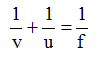# Physics

### Chapter : 4. Light Refraction

#### Important Points To Be Remember (Light Refraction)

Important Points To Be Remember
Refraction : The bending of a ray of light as it passes from one medium to another is called refraction.
(a) A ray of light travelling from a rarer medium to denser medium (say, water or glass) bends towards the normal.
(b) A ray of light travelling from a denser to a rarer medium bends (or refractive) away from the normal.
Laws of refraction :
(a) The ratio of sine of the angle of incidence to the sine of the angle of refraction for a particular pair of media is constant, i.e. sin i / sin r = constant is equal to the refractive index of the medium into which the light is entering.
(b) The incident ray, the refracted ray and the normal all lie in the same plane.
Lateral displacement : The perpendicular distance of separation between the emergent ray and the original path of the incident ray is called lateral displacement.
Lens : A piece of any transparent material bound by two curved surfaces is called a lens. A lens which is thicker in the middle and thinner at the edges is called a convex lens. A convex lens is also called converging lens.
A lens which is thicker at the edges and thinner at the centre is called a concave lens.
A concave lens is also called a diverging lens.
Optical centre of a lens : The centre point of a lens is called its optical centre. A ray of light passing through the optical centre does not suffer any deviation.
Image formed by the lenses : A convex lens forms real and inverted images for all the positions of an object at and outside the focus (F). However, when the object is placed between F and O, the image formed by a convex lens is virtual and erect.
A concave lens always forms a virtual, erect and a diminished image whatever may be the distance of the object from the lens.
Lens formula :
The lens formula isPower of a lens : Reciprocal of the focal length of a lens measured in metres is called its power. Power of a lens is described in dioptre (D) unit.
Refractive indices of various substances relative to vacuum with light of wavelength 589 mm
Solids (at 20ºC)
Substance Refractive index
Diamond 2.42
Ruby 1.71
Sapphire 1.77
Quartz (fused) 1.46
Rock salt 1.54
Glass (crown) 1.52
Glass (flint) 1.66
Ice 1.31

Liquids (at 20ºC)
Substance Refractive index
Water 1.33
Ethyl Alcohol 1.36
Kerosene 1.44
Turpentine Oil 1.47
Glycerine 1.47
Benzene 1.5
Carbon Disulphide 1.63

Gases (at 0ºC, 1 atm)
Substance Refractive index
Air 1.00029
Carbon dioxide 1.00045